Question

# 1. You can swing a ball on a string in a vertical circle if you swing...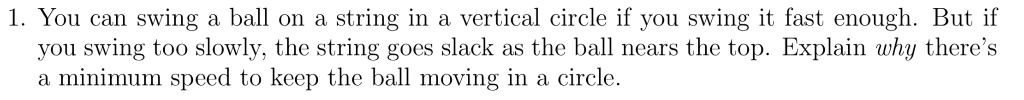1. You can swing a ball on a string in a vertical circle if you swing it fast enough. But if you swing too slowly, the string goes slack as the ball nears the top. Explain why there's a minimum speed to keep the ball moving in a circle.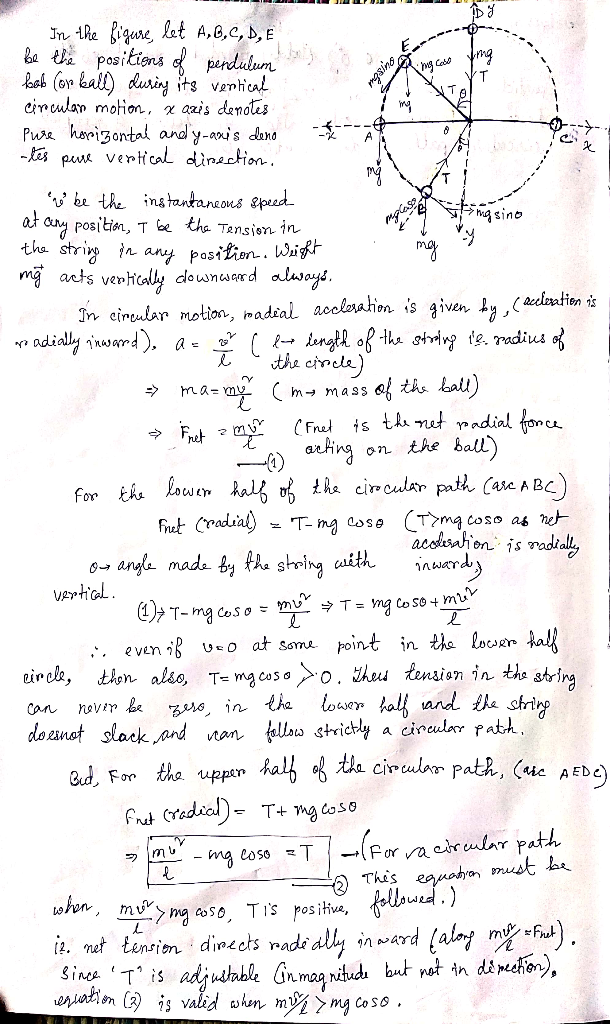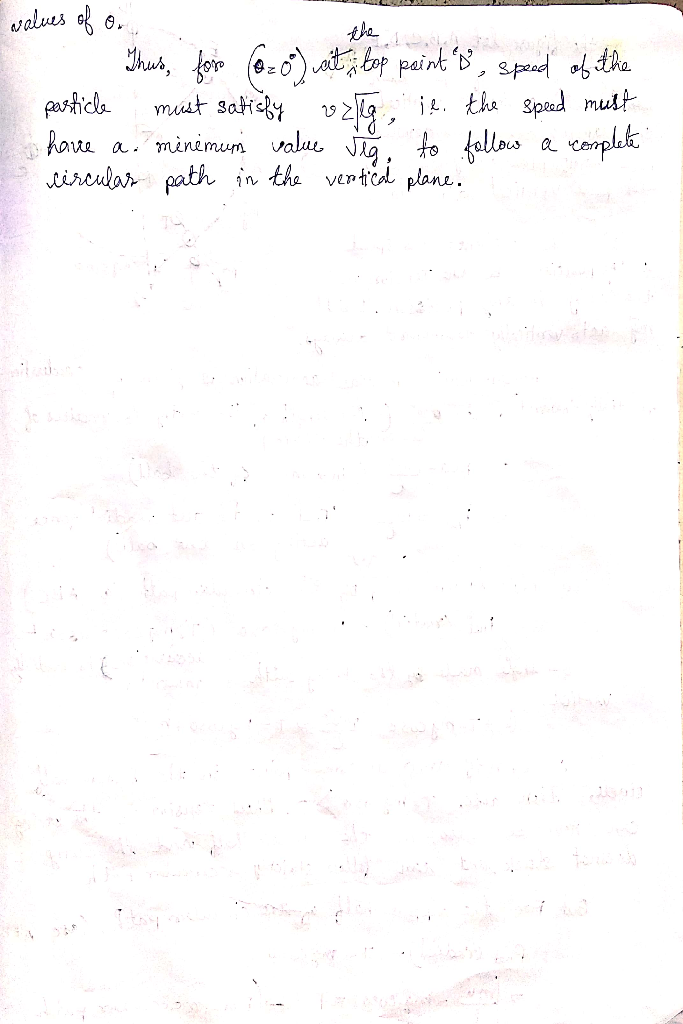#### Earn Coins

Coins can be redeemed for fabulous gifts.

Similar Homework Help Questions

You can swing a ball on a string in a vertical circle if you swing it fast enough. But if you swing too slowly, the string goes slack as the ball nears the top.Explain why there's a minimum speed to keep the ball moving in a circle.

• ### Suppose you swing a ball of mass m in a vertical circle on a string of...

Suppose you swing a ball of mass m in a vertical circle on a string of length L. As you probably know from experience, there is a minimum angular velocity ωmin you must maintain if you want the ball to complete the full circle without the string going slack at the top. Find an expression for ωmin in terms of g and L . Evaluate ωmin in rpm for a 65 g ball tied to a 1.4-m-long string.

• ### A ball of mass m, at one end of a string of length L, rotates in a vertical circle just fast...

A ball of mass m, at one end of a string of length L, rotates in a vertical circle just fast enough toprevent the string from going slack at the top of the circle. The speed of the ball at the bottom of thecircle is:The answer says square root (5gL) where L is the length of the string..I get square root (4gL) using conservation of energy.

• ### You swing a ball in a vertical circle at the end of a string that always...

You swing a ball in a vertical circle at the end of a string that always remains taut. At the top of the circle, the centripetal force on the ball is a.)larger thant its weight,b.)onehalf of its weight c) smaller than its weight d.) equal to its weight d.) twice its weight

• ### ball on a string

5. A ball of mass m, at one end of a string of length L, rotates in a vertical circle just fast enough to prevent the string from going slack at the top of the circle.The speed of the ball at the bottom of the circle is:

• ### A tennis ball connected to a string is spun around in a vertical, circular path at...A tennis ball connected to a string is spun around in a vertical, circular path at a uniform speed. The ball has a mass m = 0.153 kg and moves at v = 5.08 m/s. The circular path has a radius of R = 0.99 m 1)What is the magnitude of the tension in the string when the ball is at the bottom of the circle? 2)What is the magnitude of the tension in the string when the ball is...

• ### A tennis ball connected to a string is spun around in a vertical, circular path at...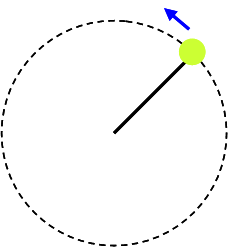A tennis ball connected to a string is spun around in a vertical, circular path at a uniform speed. The ball has a mass m = 0.175 kg and moves at v = 5.22 m/s. The circular path has a radius of R = 1.14 m 1.What is the magnitude of the tension in the string when the ball is at the bottom of the circle? 2.What is the magnitude of the tension in the string when the ball is...

• ### A tennis ball connected to a string is spun around in a vertical, circular path at...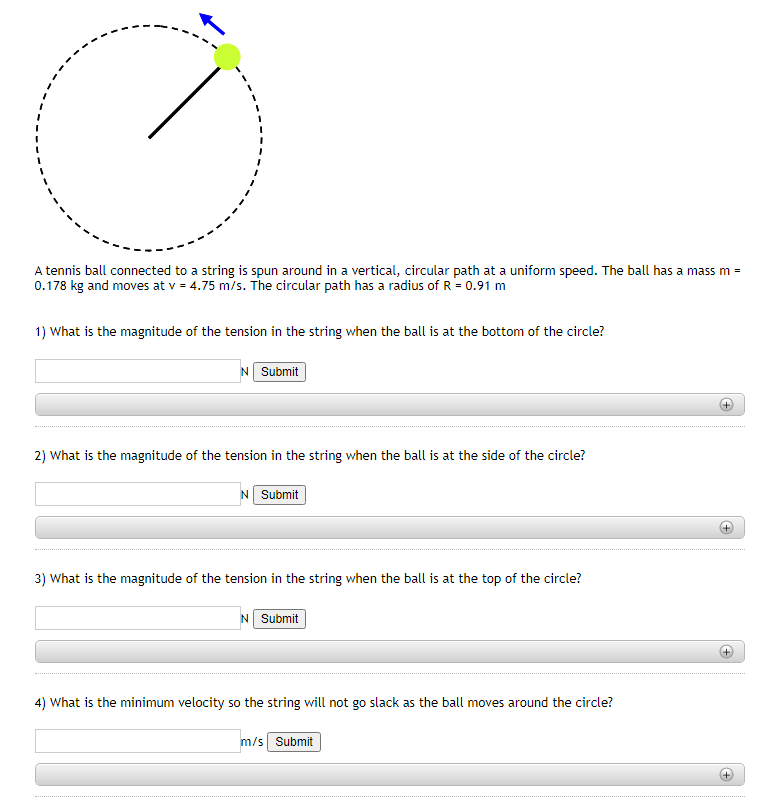A tennis ball connected to a string is spun around in a vertical, circular path at a uniform speed. The ball has a mass m = 0.178 kg and moves at v = 4.75 m/s. The circular path has a radius of R = 0.91 m 1) What is the magnitude of the tension in the string when the ball is at the bottom of the circle? N Submit + 2) What is the magnitude of the tension in the...

• ### - 13. A ball attached to a string of length r rotates in a vertical circle....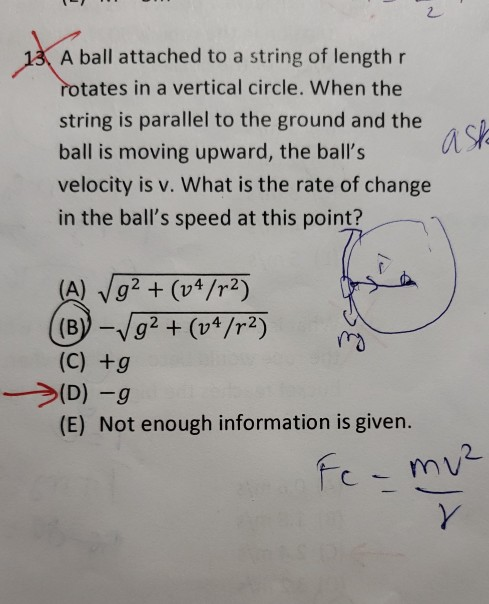- 13. A ball attached to a string of length r rotates in a vertical circle. When the string is parallel to the ground and the ball is moving upward, the ball's velocity is v. What is the rate of change in the ball's speed at this point? asla (A) Vg2 + (04/r2) ((B)) - √g²+ (v4/72) (C) +g (D)-g (E) Not enough information is given. Fc-my²

• ### A 900 g ball moves in a vertical circle on a 1.07 m -long string. If...

A 900 g ball moves in a vertical circle on a 1.07 m -long string. If the speed at the top is 4.10 m/s , then the speed at the bottom will be 7.67 m/s . A) What is the ball's weight? B) What is the tension in the string when the ball is at the top? C) What is the tension in the string when the ball is at the bottom?